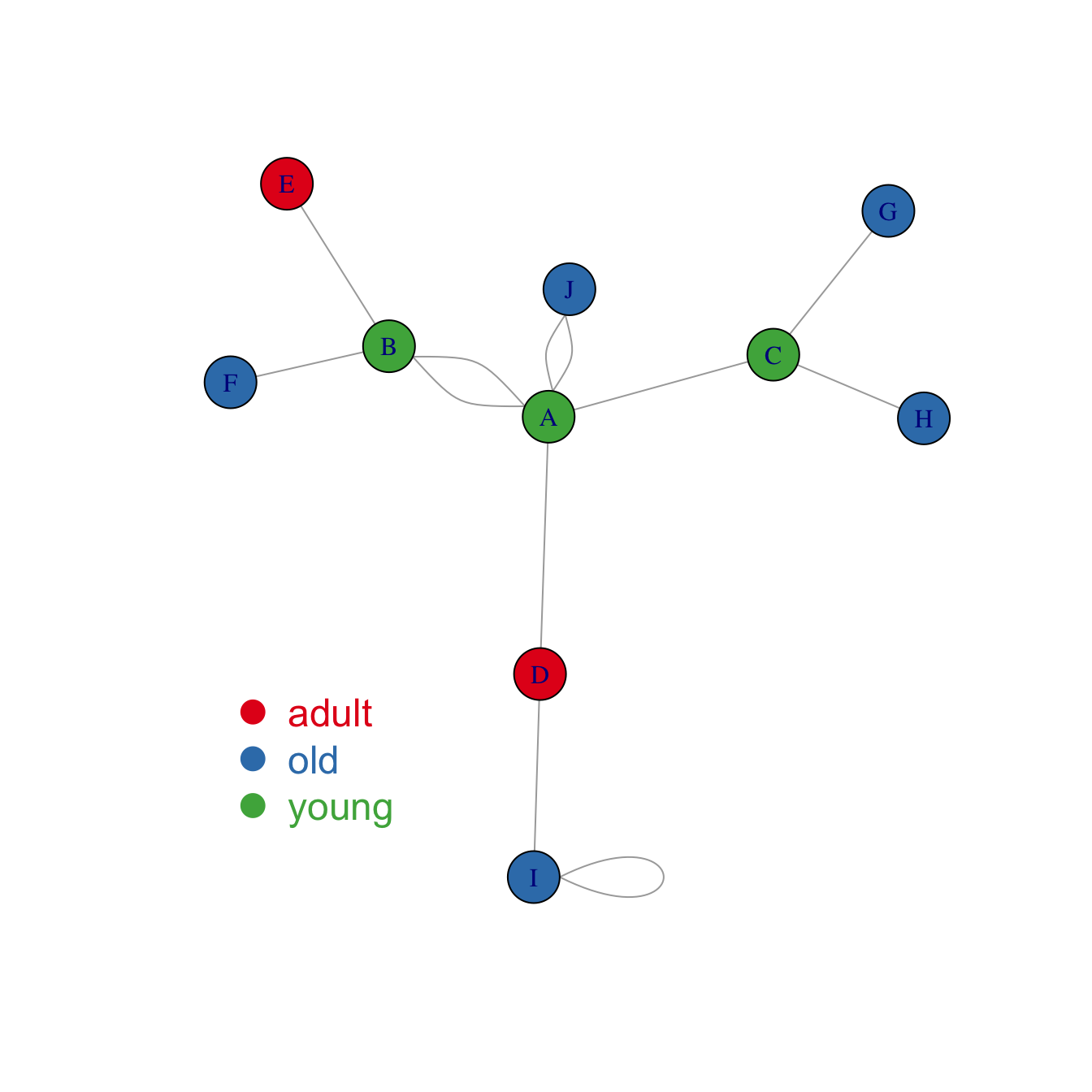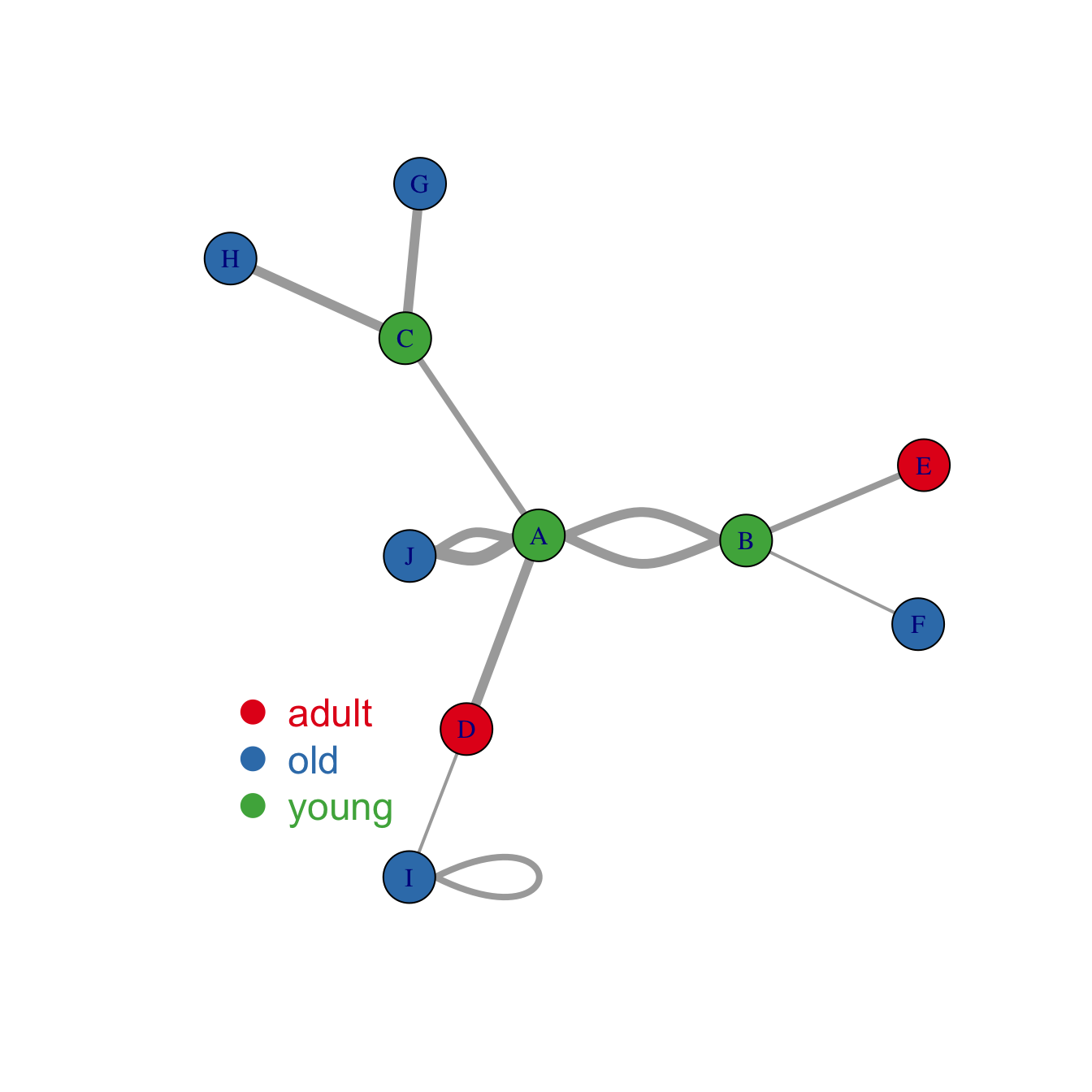# Map variable to node and links feature in R igraph network

The `igraph` package is the best way to build network diagram with R. This post shows how to map a variable to node or link features, allowing to add more insight to the chart.

# Map variable to node features

Once you know how to make a basic network graph and how to customize its general features, you probably want to map the features according to another variable.

Here we consider a network with 10 people. Each is either adult, old or young and we want one specific color for each category.

The dataset is composed by 2 data frames. `Links` provides the links between people. `Nodes` gives features concerning people. What we need is to transform the `carac` column into a vector of 3 colors, and provide this vector to the plot. The 3 colors are picked up in an `Rcolorbrewer` palette as described in graph #39.``````# library
library(igraph)

# create data:
source=c("A","A", "A", "A", "A","J", "B", "B", "C", "C", "D","I"),
target=c("B","B", "C", "D", "J","A","E", "F", "G", "H", "I","I"),
importance=(sample(1:4, 12, replace=T))
)
nodes <- data.frame(
name=LETTERS[1:10],
)

# Turn it into igraph object

# Make a palette of 3 colors
library(RColorBrewer)
coul  <- brewer.pal(3, "Set1")

# Create a vector of color
my_color <- coul[as.numeric(as.factor(V(network)\$carac))]

# Make the plot
plot(network, vertex.color=my_color)

legend("bottomleft", legend=levels(as.factor(V(network)\$carac))  , col = coul , bty = "n", pch=20 , pt.cex = 3, cex = 1.5, text.col=coul , horiz = FALSE, inset = c(0.1, 0.1))``````

# Map variable to link features

Following the same principle, it is possible to map other variables to other parameters.

Here is an example where we map the importance of the nodes to the edge width. (There is an `importance` column in the `links` data frame)``````# Check
#E(network)\$importance

# Plot
plot(network, vertex.color=my_color, edge.width=E(network)\$importance*2 )
legend("bottomleft", legend=levels(as.factor(V(network)\$carac))  , col = coul , bty = "n", pch=20 , pt.cex = 3, cex = 1.5, text.col=coul , horiz = FALSE, inset = c(0.1, 0.1))``````

Related chart types

## Contact

This document is a work by Yan Holtz. Any feedback is highly encouraged. You can fill an issue on Github, drop me a message on Twitter, or send an email pasting yan.holtz.data with gmail.com.